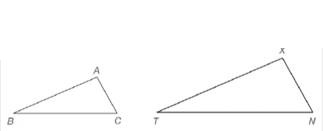Chapter 5.2, Problem 6EElementary Geometry for College St...

6th Edition
Daniel C. Alexander + 1 other
ISBN: 9781285195698

Solutions

Chapter
SectionElementary Geometry for College St...

6th Edition
Daniel C. Alexander + 1 other
ISBN: 9781285195698
Textbook Problem

In Exercises 5 and 6, refer to the drawing.a) If △ A B C ∼ △ X T N , which angle of △ A B C corresponds to ∠ N of △ X T N ? b) If △ A B C ∼ △ X T N , which side of △ X T N corresponds to side A C ¯ of △ A B C ?To determine

a)

To find:

The angle in ΔABC that corresponds to N of ΔXTN.

Explanation

Definition used:

Similarity between Two polygons:

Two polygons are similar if and only if when all pairs of corresponding angles are congruent and all pairs of corresponding sides are proportional.

Given:

The triangles ABC and XTN are similar.

Calculation:

We have ΔABCΔXTN.

Thus the corresponding angles are congruent.

That is AX,BT,CN

To determine

b)

To find:

The side in ΔXTN that corresponds to side AC¯ of ΔABC.

Still sussing out bartleby?

Check out a sample textbook solution.

See a sample solution

The Solution to Your Study Problems

Bartleby provides explanations to thousands of textbook problems written by our experts, many with advanced degrees!

Get Started

let f(x) = x3 + 5, g(x) = x2 2, and h(x) = 2x + 4. Find the rule for each function. 6. fgh

Applied Calculus for the Managerial, Life, and Social Sciences: A Brief Approach

Convert the expressions in Exercises 6584 to power form. 1(x2+1)334(x2+1)3

Finite Mathematics and Applied Calculus (MindTap Course List)

Using for |x| < 1,

Study Guide for Stewart's Single Variable Calculus: Early Transcendentals, 8th